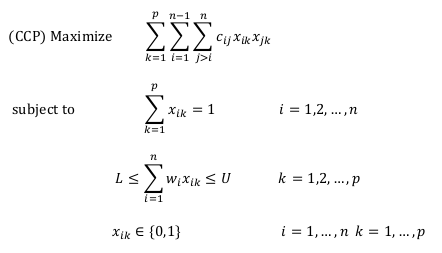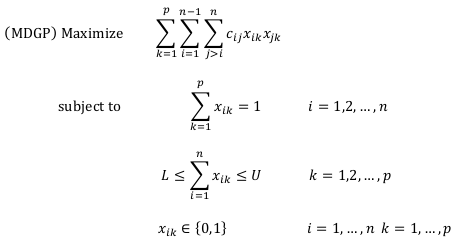# Capacitated Clustering Problem

Martínez-Gavara, Campos, Gallego, Laguna and Martí (2013)
Optsicom project, University of Valencia (Spain)

# Problem Description

The Capacitated Clustering Problem (CCP) consists of forming a specified number of clusters or groups from a set of elements in such a way that the sum of the weights of the elements in each cluster is within some capacity limits, and the sum of the benefits between the pairs of elements in the same cluster is maximized. This problem arises in the context of facility planners at mail processing and distribution. The Maximally Diverse Grouping Problem (MDGP) is a special case of the CCP in which all the elements have a weight of one unit.

Given a graph G = (V, E) where V is a set of n nodes and E is a set of edges, let wi ≥ 0 be the weight of node iV and let cij be the benefit of edge (i, j)E. The Capacitated Clustering Problem (CCP) consists of partition V into p clusters in such a way that the sum of the weights of the elements in each cluster is within some integer capacity limits, L and U, and the sum of the benefits between the pairs of elements in the same cluster is maximized.

The CCP can be formulated as a quadratic integer program with binary variables xik that take the value of 1 if element i is in cluster k and 0 otherwise.The objective function adds the total benefit of all pairs of elements that belong to the same cluster. The first set of constraints forces the assignment of each element to a cluster. The second set of constraints forces the sum of the weights of the pairs of elements in the same cluster to be between L and U.

The literature on the CCP and related problems is vast and a recent paper (Deng and Bard, 2011) summarizes previous heuristics and formulations for this problem.

The maximally diverse grouping problem (MDGP) consists of grouping a set of elements into p mutually disjoint groups in such a way that the diversity among the elements in each group is maximized. The diversity among the elements in a group is calculated as the sum of the individual distance between each pair of elements. The objective of the problem is to maximize the overall diversity, i.e., the sum of the diversity of all groups, when the size of each group is within a specified range. Clearly, the MDGP is a special case of the CCP for which wi = 1 for all node i, and the distance between each pair of nodes (i, j) is the benefit cij. Therefore, from the CCP formulation above, the MDGP can be formulated as:The MDGP is called the k-partition problem in Feo et al. (1992) and the equitable partition problem in O’Brien and Mingers (1995). One of the most popular MDGP applications appears in the academic context of forming student groups (Weitz and Jelassi, 1992).

# State of the Art Methods

The most relevant approximated methods developed to solve this problem are:

• Prev_GRASP: The GRASP method by Deng and Bard (2011).
• AdTS_SO: Adaptation of the tabu search by Gallego et al. (2013).
• PR_HMP: GRASP with Path Relinking by Morán-Mirabal et al. (2013) for the handover minimization problem.
• GRASP: Proposed GRASP method.
• TS: Proposed Tabu search method.
• GRASP+TS: A combination of the GRASP and TS methods. The GRASP+TS hybrid consists of running GRASP for half of the total running time in the experiment, and then, for the rest of the running time, applying TS starting from the best solution found by GRASP.

# Instances (CCPLIB)

We employed 50 instances in our experimentation. This benchmark set of instances is referred to as CCPLIB. The set is divided into two subsets:

• RanReal: This set, originally proposed by Gallego et al. (2013) for the MDGP, consists of 40 n × n matrices in which the benefit values cij are real numbers generated using a Uniform distribution U(0,1000). We have adapted these instances to the CCP by generating the node weights with a Uniform distribution U(0,10). There are 20 instances with n = 240, p = 12, L = 75, and U = 125. The remaining 20 instances have n = 480, p = 20, L = 100, and U = 150.
• DB: This set is based on the problem with n = 82 and p = 8 introduced by Deng and Bard (2011) in the context of mail delivery. We keep the benefit values as they appear in that original instances. However, instead of considering all the node weights equal to 1, as in Deng’s and Bard’s article, we randomly generate the node weights with a Uniform distribution U(0,10) and thus changing the instances from MDGP to CCP. The set consists of 10 instances with n = 82, p = 8, L = 25, and U = 75.
• MM: The benchmark set of 83 synthetic instances introduced in Morán-Mirabal et al. (2013) for the handover minimization problem. It consists of five instances for each of the following combinations (b,r) of number of base stations and RNCs: (20, 5), (30, 5), (30, 10), (40, 5), (40, 10), (40, 15), (100, 15), (100, 25), (100, 50), (200, 15), (200, 25), (200, 50), (400, 15), (400, 25), (400, 50), and four instances with the following combinations: (20, 10), (30, 15).

# Computational Experience

We have performed a computational comparison of the state of the art methods on all of the 50 instances. We run each method for 60 seconds on each instance.

The methods Prev_GRASP and AdTS_SO were implemented in Java SE 6. The methods GRASP and TS were implemented in C. All experiments were conducted on an Intel Core 2 Quad CPU Q 8300 with 6 GB of RAM.

In this experiment we compute for each instance and each method the relative deviation Dev (in percent) between the best solution value, Value, obtained with the method and the best known value of this instance, BestValue. For each method, we also report the number of instances #Best for which the method obtains the best value.

In this experiment we compute, for each method, the average percent deviation from the best known solutions (Dev) and the number of instances in which the method is able to match the best solutions obtained (out of 50 instances) (#Best). We also compute the Score statistic described in Ribeiro et al. (2002) (Score). For each problem instance in the experiment, the Score of a method is the number of methods that found a solution that is strictly better than the one that the method being scored found. For a set of instances, the Score is the sum of the individual scores.

Method Dev #Best Score
Prev_GRASP 9.43% 0 79
TS 0.15% 16 4
GRASP 14.55% 0 100
GRASP+TS 0.61% 4 16
Table 1. Comparison of best methods on the 20 RanReal instances with n = 240
Method Dev #Best Score
Prev_GRASP 41.78% 0 100
TS 1.99% 10 10
GRASP 19.85% 0 80
GRASP+TS 2.07% 10 13
Table 2. Comparison of best methods on the 20 RanReal instances with n = 480
Method Dev #Best Score
Prev_GRASP 0.33% 0 41
TS 4.52% 0 50
GRASP 0.08% 2 20
GRASP+TS 0.13% 2 21
Table 3. Comparison of best methods on the 10 DB instances

In the last experiment, we compare the previous algorithm GRASP with Path Relinking for the handover minimization problem, PR_HMP, with the two best methods identified in the previous experiments: AdTS_SO and GRASP+TS. We apply these three methods to solve the 83 instances in the MM set. As in the previous experiments, we run each method for 60 seconds on each instance. We have also run PR_HMP method for 15 minutes. Table 4 shows the associated results in which we add to the metrics reported in the previous experiments, the running time to the best solution that each method is able to find (Time to best).

Method Dev #Best Score Time to best
PRM_HMP(60 s.) 2.5% 39 125 19.2
PR_HMP(15 m.) 1.6% 40 78 256.6
AdTS_SO(60 s.) 0.1% 79 14 42.9
GRASP+TS(60 s.) 0.3% 53 33 14.8
Table 4. Comparison of best methods on the 83 MM instances

# References

• Deng, Y. and J.F. Bard (2011) “A reactive GRASP with path relinking for the capacitated clustering,” Journal of Heuristics, vol. 17, pp. 119-152.
• Feo, T., O. Goldschmidt and M. Khellaf (1992) “One-half approximation algorithms for the k-partition problem,” Operations Research, vol. 40, pp. S170-S173.
• Gallego, M., M. Laguna, R., Martí, and A. Duarte (2013) “Tabu search with strategic oscillation for the maximally diverse grouping problem,” Journal of the Operational Research Society, vol. 64, no. 5, pp. 724–734.
• Morán-Mirabal, L. F., J. L. González-Velarde, M. G. C. Resende, and R. M. A. Silva (2013) “Randomized heuristics for handover minimization in mobility networks,” Journal of Heuristics, vol. 19, pp. 845-880.
• O’Brien, F. A. and J. Mingers (1995) “The equitable partitioning problem: a heuristic algorithm applied to the allocation of university student accommodation,” Warwick Business School, Research Paper no. 187.
• Weitz, R. R. and M. T. Jelassi (1992) “Assigning students to groups: a multi-criteria decision support system approach,” Decision Sciences, vol. 23, no. 3, pp. 746-757.